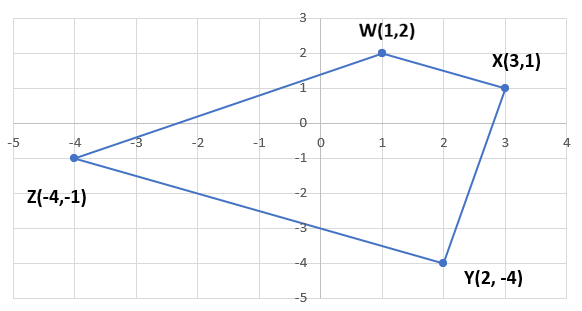A Quadrilaterals are identified as 4-sided polygons, whose interior angles always add up to $$360°$$.
There are 5 distinct types of quadrilaterals, each with their own unique sets of features: Rectangles, Squares, Parallelograms, Rhombuses, and Trapezoids.

### Square

• Contains 4 equal sides, all of which are parallel to each other
• Has 4 right angles, meaning that each angle is $$90°$$
• Diagonals bisect each other perpendicularly

### Rectangle

• Contains 2 pairs of adjacent sides, each of which are equal and parallel to each other
• Has 4 right angles, meaning that each angle is $$90°$$
• Diagonals bisect each other

### Parallelogram

• Are formed within the midsegments of any quadrilateral
• Contains 2 pairs of adjacent sides both of which are parallel and equal to each other
• Opposite angles are equal to each other
• Diagonals bisect each other

### Rhombus

• All 4 sides are equal
• Contains 2 pairs of adjacent sides both of which are parallel and equal to each other
• Diagonals bisect each other perpendicularly

### Trapezoid

• Has only one pair of parallel sides, referred to as bases
• Contains a pair of lateral sides, which are positioned at an angle and not parallel to each other
• The midsegment is parallel to the parallel sides; its length is the average of those sides

What is the midlength of the following Trapezoid?For a quadrilateral $$\text{STUV}$$ with vertices $$\text{S}(-2, 4)$$, $$\text{T}(-4,-2)$$, $$\text{U}(2,-4)$$, and $$\text{V}(4,0)$$:
1. Find the midpoint $$\text{D}$$ of side $$\text{ST}$$, midpoint $$\text{E}$$ of side $$\text{TU}$$, midpoint $$\text{F}$$ of side $$\text{UV}$$, and midpoint $$\text{G}$$ of side $$\text{VS}$$.
2. Verify that opposite sides of $$\text{DEFG}$$ are parallel and equal in length.
3. What type of shape is $$\text{DEFG}$$?
4. Draw the $$\text{STUV}$$ quadrilateral and join the midpoints of adjacent sides to form a new quadrilateral DEFG to check your answer.